# Important questions with Answer of Alternating current For Jee Main And Advanced examination

The circuit is shown below.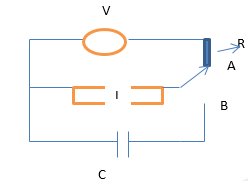Currently the switch is in position A and steady current is flowing to the circuit. Capacitor is uncharged. At t=0, switch is instanously moved to position B.
Question 1 Find the current in the LC circuit for t≥ 0
Here $\omega = \frac {1} {\sqrt LC}$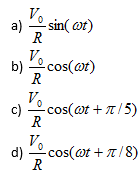Question 2 Find the charge q on the lower capacitor plate for t≥ 0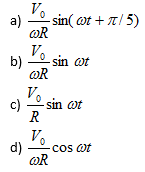Question 3 Which of the following is true of the circuit?
a) The electric oscillations of time period $2\pi \sqrt{LC}$ are produced in the LC circuit
b) The total energy remains conserved and is equal to $\frac {1}{2}L \frac {V_0^2}{R}$
c) The maximum current in the circuit is V0/R
d) None of these

Question 4
An LR circuit is connect to Voltage source V0. Which of the following is true at time equal to time constant
a) The rate at which energy is being stored in Inductor is given by $\frac {.63 V_0^2}{R}$
b) Power loss in the resistor is given by $\frac {.40 V_0^2}{R}$
c) The rate of change of current is $\frac {.37 V_0^2}{R}$
d) Current is 63% of the maximum value

Question 5
A circuit is shown below in Figure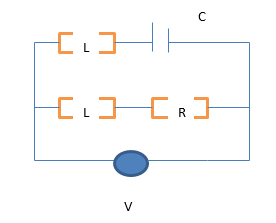It is given
L=R2C
Which of the following is true
a) The total current will instantly rise to its final value V0/R when switch is closed
b) The current will zero in LR branch and have value V0/R in the LC circuit path at t=0
c) The current will rise with time in the LR circuit and decrease with time in LC circuit
d) Total current in the circuit will always be V0/R for t>0

A sinusoidal voltage of frequency f=60Hz and peak value 150V is applied to a series LR circuit
Where L=40mH and R=20ohm
Question 6 Which one of the following is correct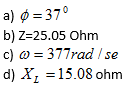Question 7 At t=T/6, which one of the following is true
a) The instantaneous current is 2.344 A
b) The instantaneous voltage across inductor is 83.29 V
c) The instantaneous voltage across resistor is 46.88 V
d) The total voltage applied is 130 V
Question 8 Which one of the following is true about the circuit?
a) Average power into the circuit is 360 W
b) Irms is 4.24 A
c) Vrms  is  106.07 V
d) None of these

Question 9:
A coil of inductance .50H and resistance 100 ohm is connected to 240 V,50Hz AC supply
Which of the following is true
a) Irms in the coil is 1.28 A
b) The time lag between voltage maximum and current maximum  is 3.2X10-3 s
c) Maximum Voltage is 339.36 V
d) None of these

Question 10 Consider a series RC circuit for which R = 2.0 × 106, C = 6.0 µF and E = 20 V. Find
(i) The time constant of the circuit,
(ii) The maximum charge on the capacitor after a switch in the circuit is closed, and
(iii) The current in the circuit at the instant just after the switch in the circuit is closed

Note to our visitors :-

Thanks for visiting our website.